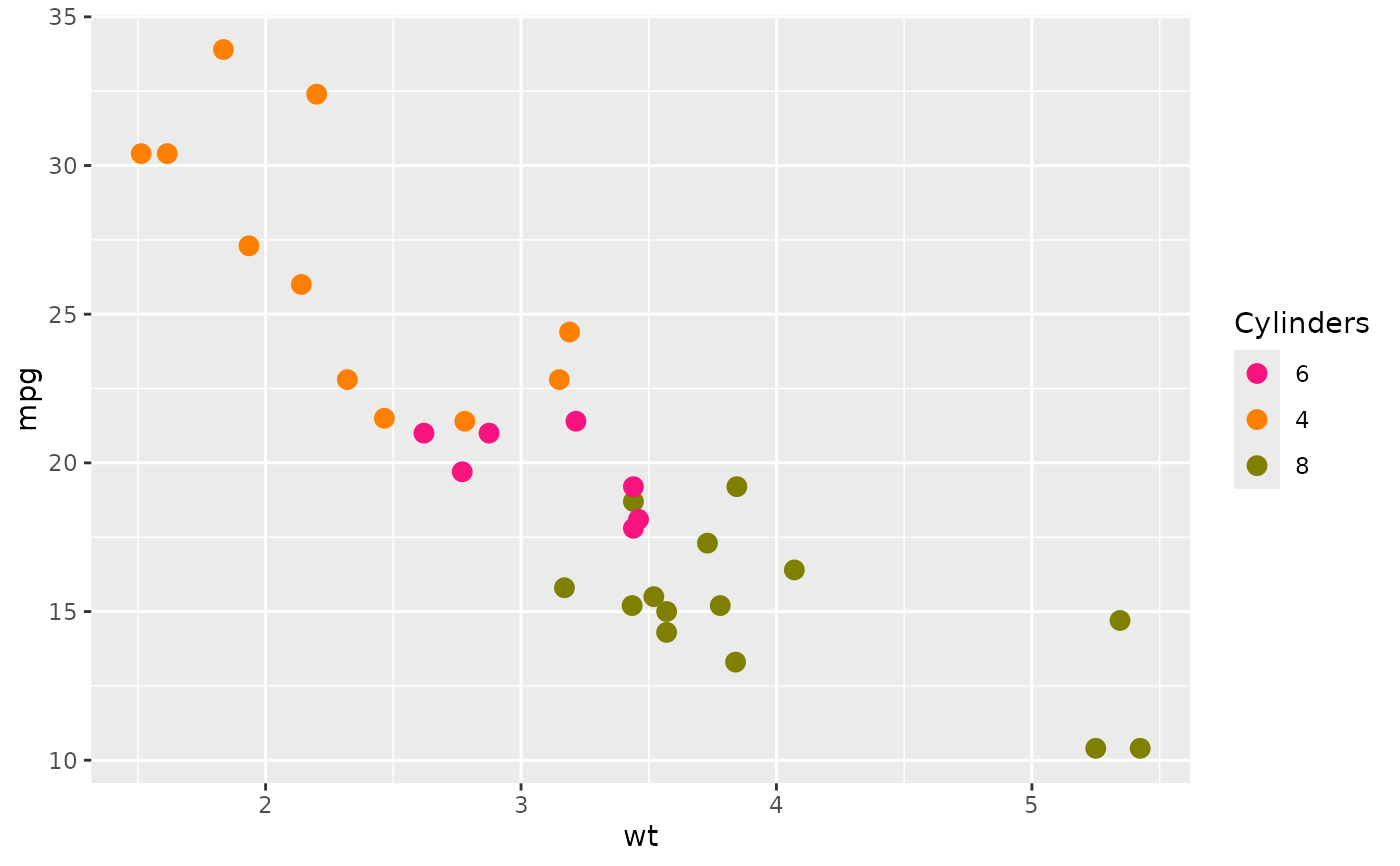A collection of discrete colour scales that use palettes which mirror the colour schemes available in GraphPad Prism.

scale_colour_prism(palette = "colors", ...)

scale_color_prism(palette = "colors", ...)

## Arguments

palette

string. Palette name, use lengths(ggprism_data\$colour_palettes) to show all valid palette names and their number of values each palette supports.

...

Arguments passed on to ggplot2::discrete_scale

name

The name of the scale. Used as the axis or legend title. If waiver(), the default, the name of the scale is taken from the first mapping used for that aesthetic. If NULL, the legend title will be omitted.

breaks

One of:

• NULL for no breaks

• waiver() for the default breaks (the scale limits)

• A character vector of breaks

• A function that takes the limits as input and returns breaks as output. Also accepts rlang lambda function notation.

labels

One of:

• NULL for no labels

• waiver() for the default labels computed by the transformation object

• A character vector giving labels (must be same length as breaks)

• An expression vector (must be the same length as breaks). See ?plotmath for details.

• A function that takes the breaks as input and returns labels as output. Also accepts rlang lambda function notation.

limits

One of:

• NULL to use the default scale values

• A character vector that defines possible values of the scale and their order

• A function that accepts the existing (automatic) values and returns new ones. Also accepts rlang lambda function notation.

expand

For position scales, a vector of range expansion constants used to add some padding around the data to ensure that they are placed some distance away from the axes. Use the convenience function expansion() to generate the values for the expand argument. The defaults are to expand the scale by 5% on each side for continuous variables, and by 0.6 units on each side for discrete variables.

na.translate

Unlike continuous scales, discrete scales can easily show missing values, and do so by default. If you want to remove missing values from a discrete scale, specify na.translate = FALSE.

na.value

If na.translate = TRUE, what aesthetic value should the missing values be displayed as? Does not apply to position scales where NA is always placed at the far right.

drop

Should unused factor levels be omitted from the scale? The default, TRUE, uses the levels that appear in the data; FALSE uses all the levels in the factor.

guide

A function used to create a guide or its name. See guides() for more information.

position

For position scales, The position of the axis. left or right for y axes, top or bottom for x axes.

super

The super class to use for the constructed scale

## Value

Returns a ggproto object of class ScaleDiscrete which works with colour aesthetics.

## Examples

library(ggplot2)

## base plot
base <- ggplot(mtcars, aes(x = wt, y = mpg, colour = factor(cyl))) +
geom_point(size = 3)

## works pretty much the same as ggplot2 scale_colour_manual
base +
scale_colour_prism(palette = "candy_bright")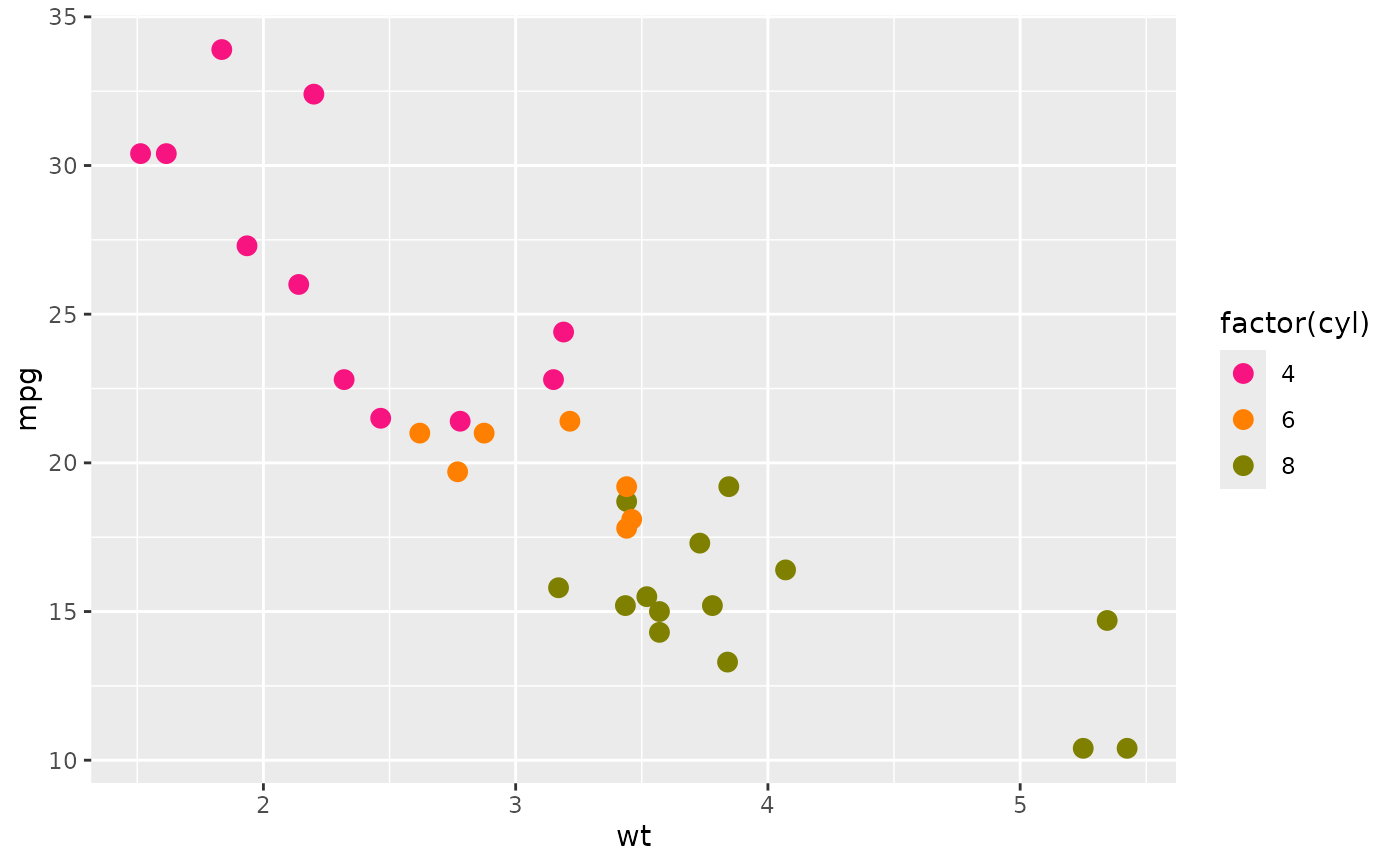## try combining the ggprism colour and fill scales
base2 <- ggplot(mpg, aes(x = class, y = hwy, fill = class, colour = class)) +
geom_boxplot()

base2 +
scale_fill_prism(palette = "floral") +
scale_colour_prism(palette = "floral")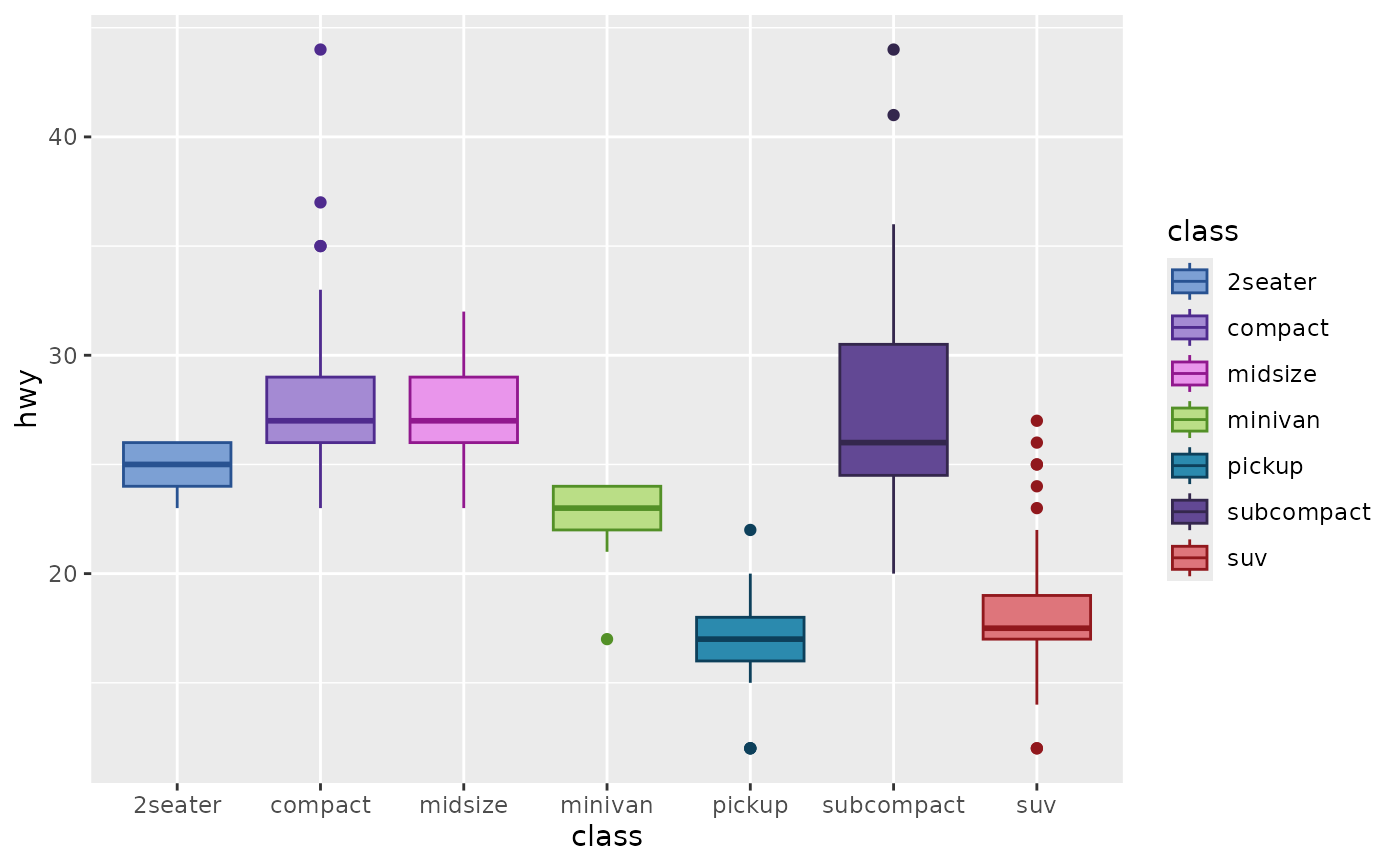## change colour scale title in legend
base +
scale_colour_prism(
palette = "candy_bright",
name = "Cylinders"
)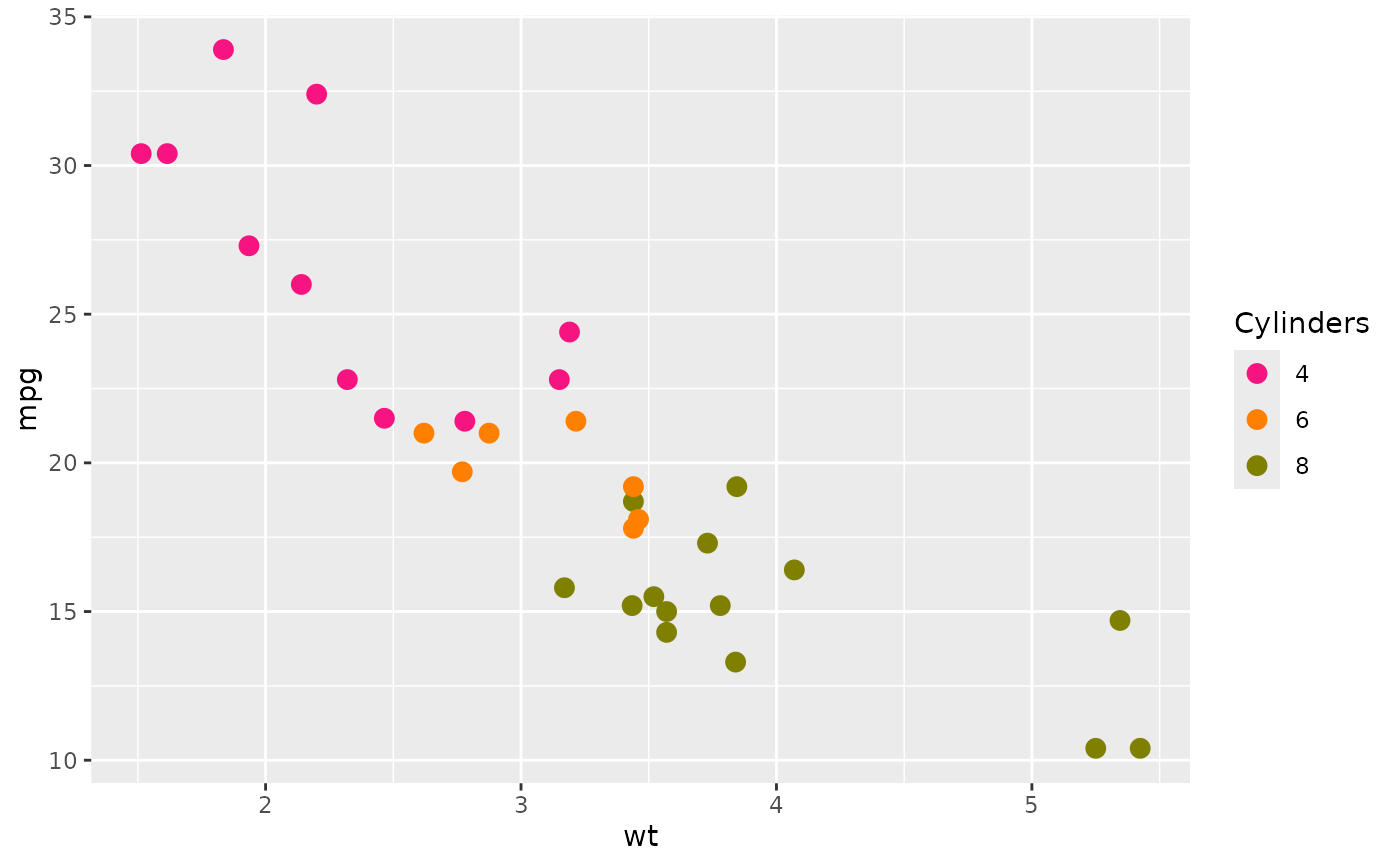## change colour labels in legend
base +
scale_colour_prism(
palette = "candy_bright",
name = "Cylinders",
label = c("4 cyl", "6 cyl", "8 cyl")
)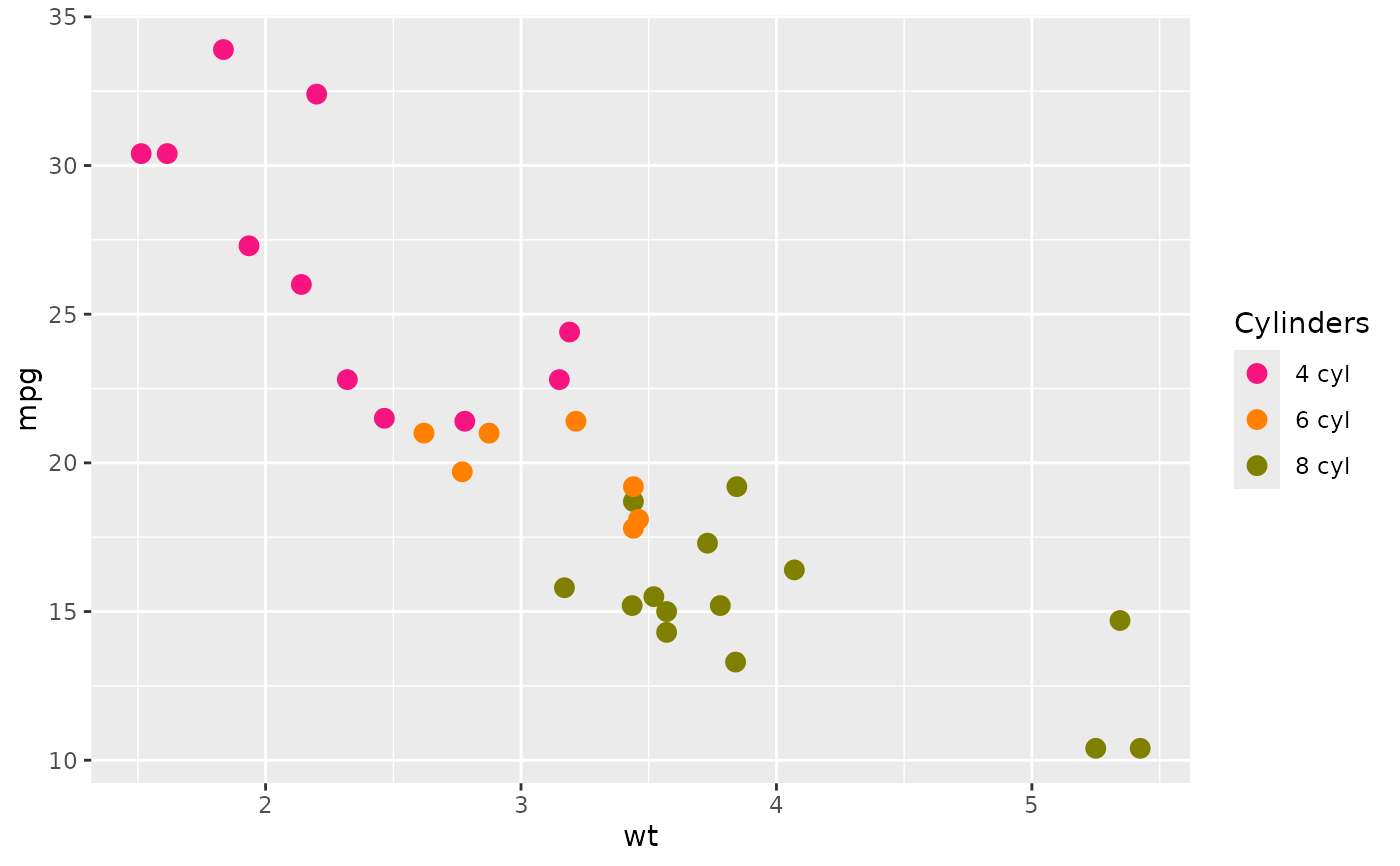## change colour labels in legend with a function
base +
scale_colour_prism(
palette = "candy_bright",
name = "Cylinders",
label = function(x) paste(x, "cyl")
)## change order of colours in legend
base +
scale_colour_prism(
palette = "candy_bright",
name = "Cylinders",
label = function(x) paste(x, "cyl"),
breaks = c(8, 4, 6)
)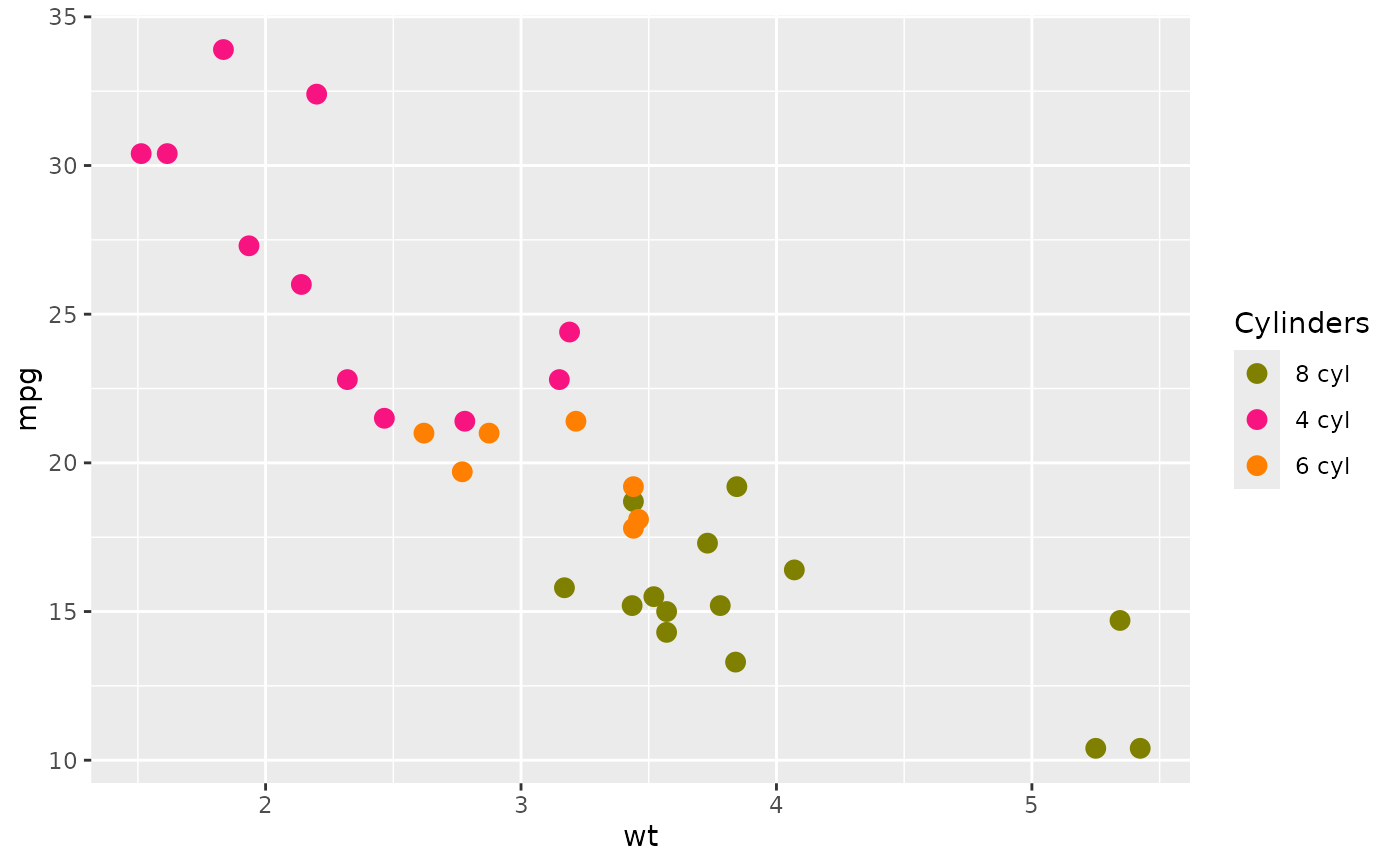## to change which colour is assigned to which cyl
## you need to change the factor levels in the underlying data
base <- ggplot(mtcars, aes(x = wt, y = mpg,
colour = factor(cyl, levels = c(6, 4, 8)))) +
geom_point(size = 3)

base +
scale_colour_prism(
palette = "candy_bright",
name = "Cylinders"
)